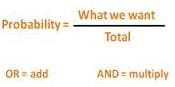# Probability - Olympiad Level MCQ, Class 10 Mathematics

## 25 Questions MCQ Test Olympiad Preparation for Class 10 | Probability - Olympiad Level MCQ, Class 10 Mathematics

Description
Attempt Probability - Olympiad Level MCQ, Class 10 Mathematics | 25 questions in 25 minutes | Mock test for Class 10 preparation | Free important questions MCQ to study Olympiad Preparation for Class 10 for Class 10 Exam | Download free PDF with solutions
QUESTION: 1

### When two coins are tossed simultaneously, what are the chances of getting at least one tail?

Solution:

At least one tails means one tails or both tails.
Let us see what the possible outcomes are when two coins are tossed simultaneouslyWe can get,
4) Tails and Tails
So we have Total = 4 outcomes.
Out of them, 3 have tails in it.
Chances of at least 1 tails = 3/4

QUESTION: 2

Solution:
QUESTION: 3

### Three fair coins are tossed simultaneously. Find the probability of getting more heads than the number of tails

Solution:

S = { HHH, HHT, HTH, HTT, THH, THT, TTH, TTT }

⇒ n(S) = 8

E = { HHH, HHT, HTH, THH }

⇒ n(E) = 4

P(E) = 4/8 = 1/2

QUESTION: 4

Three fair coins are tossed simultaneously. Find the probability of Getting one tail

Solution:
QUESTION: 5

Three fair coins are tossed simultaneously. Find the probability of Getting atleast one head

Solution:
QUESTION: 6

Three fair coins are tossed simultaneously. Find the probability of Getting two heads

Solution:
QUESTION: 7

Three fair coins are tossed simultaneously. Find the probability of Getting atleast two heads

Solution:
QUESTION: 8

Three fair coins are tossed simultaneously. Find the probability of Getting atleast one head and one tail

Solution:
QUESTION: 9

Three fair coins are tossed simultaneously. Find the probability of Getting more heads than the number of tails

Solution:
QUESTION: 10

An unbiased die is rolled. Find the probability of Getting a number less than 7 but greater than 0.

Solution:
QUESTION: 11

An unbiased die is rolled. Find the probability Getting a multiple of 3.

Solution:
QUESTION: 12

An unbiased die is rolled. Find the probability of Getting a prime number.

Solution:
QUESTION: 13

An unbiased die is rolled. Find the probability of Getting an even number.

Solution:
QUESTION: 14

A coin is tossed successively three times. Find the probability of Getting exactly one head or two heads.

Solution:
QUESTION: 15

A coin is tossed successively three times. Find the probability of Getting no heads.

Solution:
QUESTION: 16

Two dice are rolled simultaneously. Find the probability of Getting a total of 9

Solution:
QUESTION: 17

Two dice are rolled simultaneously. Find the probability of Getting a sum greater than 9.

Solution:
QUESTION: 18

Two dice are rolled simultaneously. Find the probability of Getting the sum of numbers on the two faces divisible by 3 or 4.

Solution:

Here n(S) = 6 x 6 = 36

E={(1,2),(1,5),(2,1),(2,4),(3,3),(3,6),(4,2),(4,5),(5,1),(5,4),(6,3) ,(6,6),(1,3),(2,2),(2,6),(3,1),(3,5), (4,4),(5,3),(6,2)}

=> n(E)=20

Required Probability n(P) = n(E)/n(S) = 20/36 = 5/9.

QUESTION: 19

Two dice are rolled simultaneously. Find the probability of Getting the sum as a prime number.

Solution:
QUESTION: 20

Two dice are rolled simultaneously. Find the probability of Getting atleast one 5.

Solution:
QUESTION: 21

One card is drawn from a pack of 52 cards, each of the 52 cards being equally likely to be drawn. Find the probability that The card drawn is black.

Solution:

Total cards n(S)=52
There are 26 black cards in a pack n(B)=26

P(E) = n(B)/n(S) = 26/52 = 1/2

QUESTION: 22

One card is drawn from a pack of 52 cards , each of the 52 cards being equally likely to be drawn. Find the probability that the card  drawn is neither a spade nor a king.

Solution:
QUESTION: 23

One card is drawn from a pack of 52 cards, each of the 52 cards being equally likely to be drawn. Find the probability that The card drawn is black and a queen.

Solution:
QUESTION: 24

One card is drawn from a pack of 52 cards, each of the 52 cards being equally likely to be drawn. Find the probability that The card drawn is either black or a queen.

Solution:
QUESTION: 25

One card is drawn from a pack of 52 cards, each of the 52 cards being equally likely to be drawn. Find the probability that the card drawn is either king or a queen.

Solution:Use Code STAYHOME200 and get INR 200 additional OFF Use Coupon Code